## 关于

1. 强化学习是什么？可以干什么？
2. 马尔科夫决策过程(MDP)的重要概念
3. 哈密顿-雅克比-贝尔曼(HJB)方程
4. 已知环境下HJB方程的求解算法：值迭代和策略迭代

1. 高中数学水平且年满18岁，部分内容需要你了解监督学习，你可以通过本教程前面的章节进行学习。
2. 如果你需要完成实践部分，需要有基本的 python 知识，你可以通过python快速入门快速了解python如何使用。

## 马尔科夫决策过程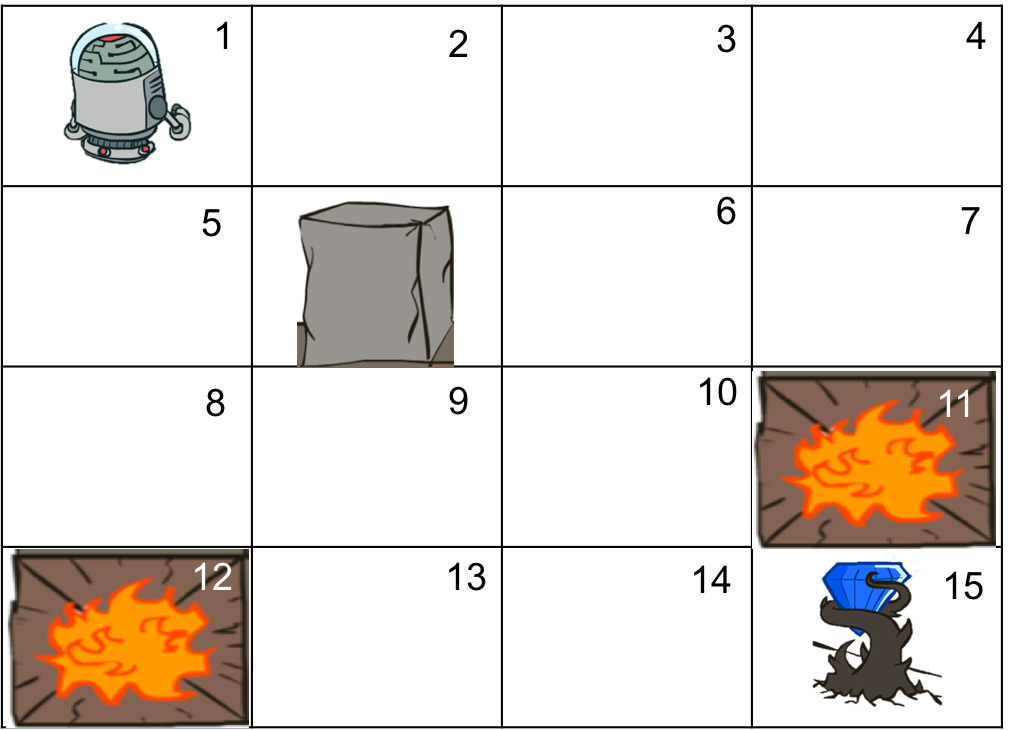1. 状态：机器人所处的位置是有限的，我们把每一个位置称作一个状态，那么一共有15个状态（有一个是石柱，机器人无法到达这个位置）。其中两个是火坑，一个是钻石，由于机器人进入这些状态就会结束游戏，我们称为终态。我们用S来表示状态，那么S可以有15个取值，我们一次用数字标识这15个状态，那么$S \in \{1,2,...,15 \}$。
2. 动作：在每个不是终态的状态下，我们都有4个控制动作，上、下、左、右，我们也可以将这些动作依次编号为1到4，用A表示动作，那么$A \in \{1,2,3,4 \}$。
3. 转移概率：因为我们是通过声音控制机器人的，所以机器人可能听错，可以认为是语音识别技术尚不成熟的原因。那么在某个状态S，采取动作A之后，机器人到达的状态并不是完全确定的。例如当机器人在左上角时，采取“右”这个动作时，机器人也有一定概率会向下移动，进入状态5（假设状态按照从左到右顺序编号）。为了描述机器人的这种不确定运动，可以用一个转移概率来表示。我们用P(S'|S, A)表示在状态S下，采取动作A的条件下，机器人进入状态S'的概率。例如在刚才这个例子中，P(S'=5|S=1, A=右)就表示在状态1（也就是左上角），采取动作右的条件下，进入状态5（也就是第二行第一个状态）的概率。所以，这个转移概率描述的是机器人所处状态在我们的控制下变化的规律。
4. 回报：在机器人每一步运动到下一个状态时，环境会给我们一个奖励或者惩罚，例如如果进入火坑游戏就会结束，而拿到钻石就会得到奖励，这种奖励或者惩罚我们用数量来量化。我们可以用正数表示奖励，负数表示惩罚，这就是回报。一般情况下，回报可能跟状态和动作都有关系，所以我们用一个函数来表示 R(S, A, S')，它表示在在状态S下采取动作A到达状态S'时，获得的回报。注意，一般情况下，回报是在每一个动作执行后跳转到新的状态就会收到的，而不是只有最后到达终点时才有。在这个例子中，只有最终的那一步才有回报，可以认为其他的每一步的回报为0。你也可以给其他的步骤给一个负的回报，负的回报可以解释为每一步付出的成本，例如时间成本、机器人的能量消耗等等。
5. 马尔科夫性：描述环境的转移概率只跟当前状态和动作有关，而与之前经过的状态和动作无关，这种性质叫做马尔科夫性（因为是一个叫马尔科夫的人最早提出来的）。在这个例子中，机器人在我们的控制下要进入的状态的概率只与当前的状态和我们的控制动作有关，而与在这之前机器人经历过的状态无关。例如，当机器人处于状态1时，那么接下来，采取动作"右"后，状态变到2还是5的概率与机器人到达状态1之间的所有事情都无关！这种无关，并不是说最终获得的回报与之前的状态无关，而是说当前这一步的转移概率与之前的状态无关！并不是所有的决策事情都有马尔科夫性的，比如股票，今天涨跌不但与昨天有关，还与之前的多天有关。如果我们用$S_t, A_t$分别代表t时刻的状态和采用的动作，那么马尔科夫性可以表示为

$$P(S_{t+1}|S_t, A_t, S_{t-1}, A_{t-1}, ...,S_1, A_1) = P(S_{t+1}|S_t, A_t)$$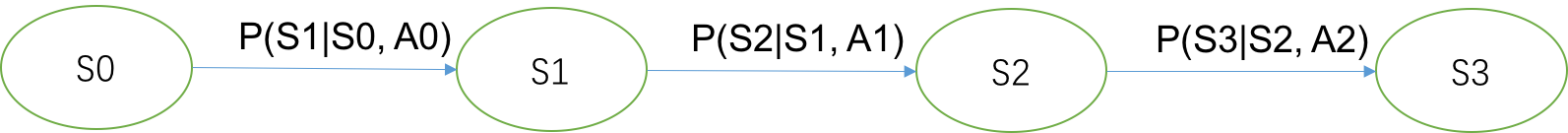$$\pi(S) = \begin{cases} \text{右}, S \in \{1,2,3,6,8,9,10,12,13,14 \} \\ \text{下}, S \in \{4,7,11 \} \end{cases}$$

MDP问题的目标是找到这样一个策略，在这个策略下，总的期望折扣回报最大化！总期望折扣回报定义如下

$$R = E \sum_{t=1}^{\infty} \gamma^{t-1} R(S_t, A_t, S' _ t), \gamma \in (0, 1]$$

### 确定性环境

$$R = 0 + 0 + 0 + 0 + 0 + 0.9^6 = 0.531$$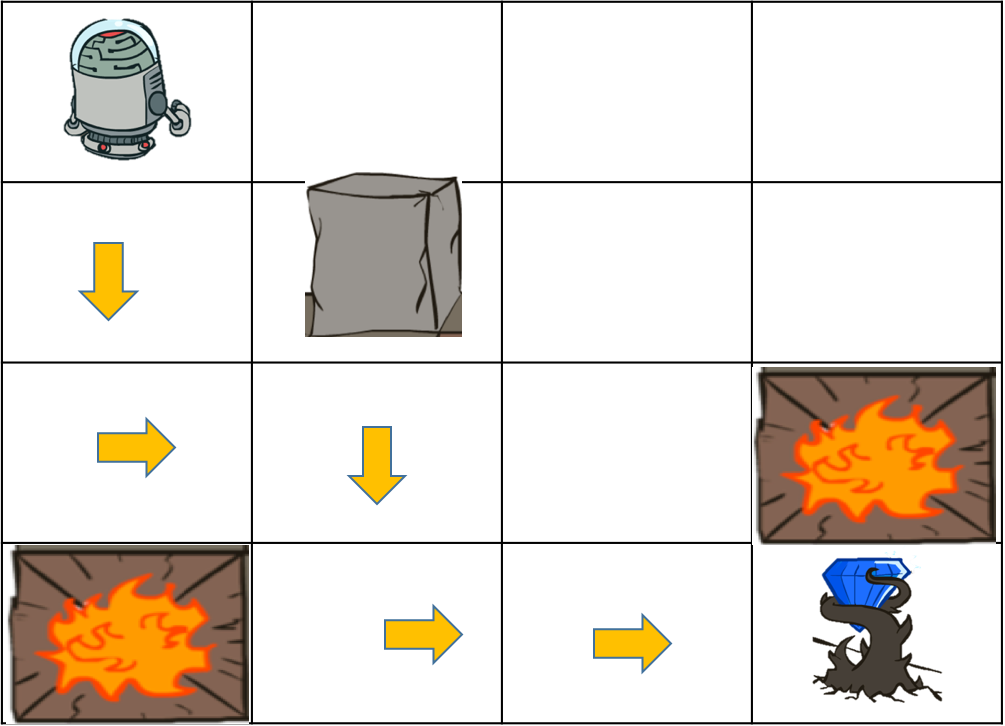## 哈密顿-雅克比-贝尔曼(HJB)方程

### 状态值函数

$$V(S) = \max E \left[ \sum_{t=1}^{\infty} \gamma^{t-1} R(S_t, A_t, S' _ t) | S_1=S \right]$$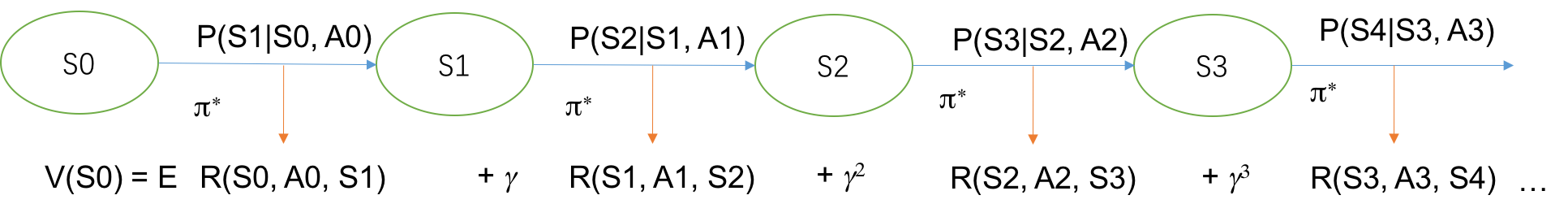$$V^{\pi}(S) = E \left[ \sum_{t=1}^{\infty} \gamma^{t-1} R(S_t, A_t, S' _ t) | S_1=S, A_t \sim \pi \right]$$

$V^{\pi}(S)$和$V(S)$都是从状态S出发获得的最大期望回报，不同的是$V^{\pi}(S)$要求后面采取的策略是给定的策略$\pi$，而$V(S)$要求的是最好的策略。假设从状态S=13出发，策略$\pi_1$是一直往上走，如果上面不能走就往右走（下图绿色箭头）。策略$\pi_2$是一直往右走，右边走不了就往下走(下图黄色箭头)。在策略1下，机器人会跳到火坑里面（这里还是认为是确定性环境，每一步回报为-0.1，火坑回报-1，钻石回报+1），所以$V^{\pi_1}(13)=-1.2$，而在策略2下，机器人将找到钻石，所以$V^{\pi_2}(13)=0.9$。显然在状态13下，策略2更好，对应的策略值函数也越大。因此，策略值函数$V^{\pi}(S)$可以用来评估一个策略好不好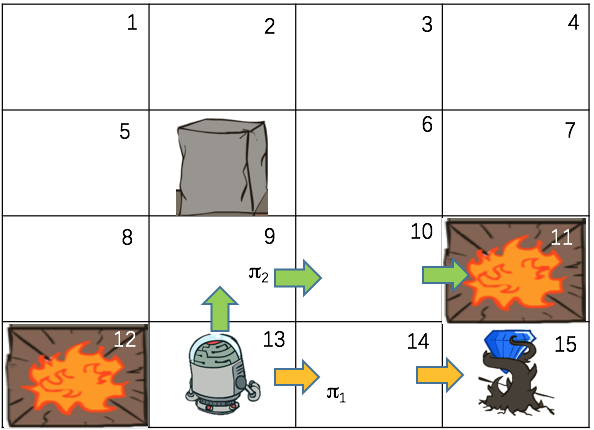### 动作值函数

$$Q(S, A) = \max E \left[ \sum_{t=1}^{\infty} \gamma^{t-1} R(S_t, A_t, S' _ t) | S_1=S, A_1=A \right]$$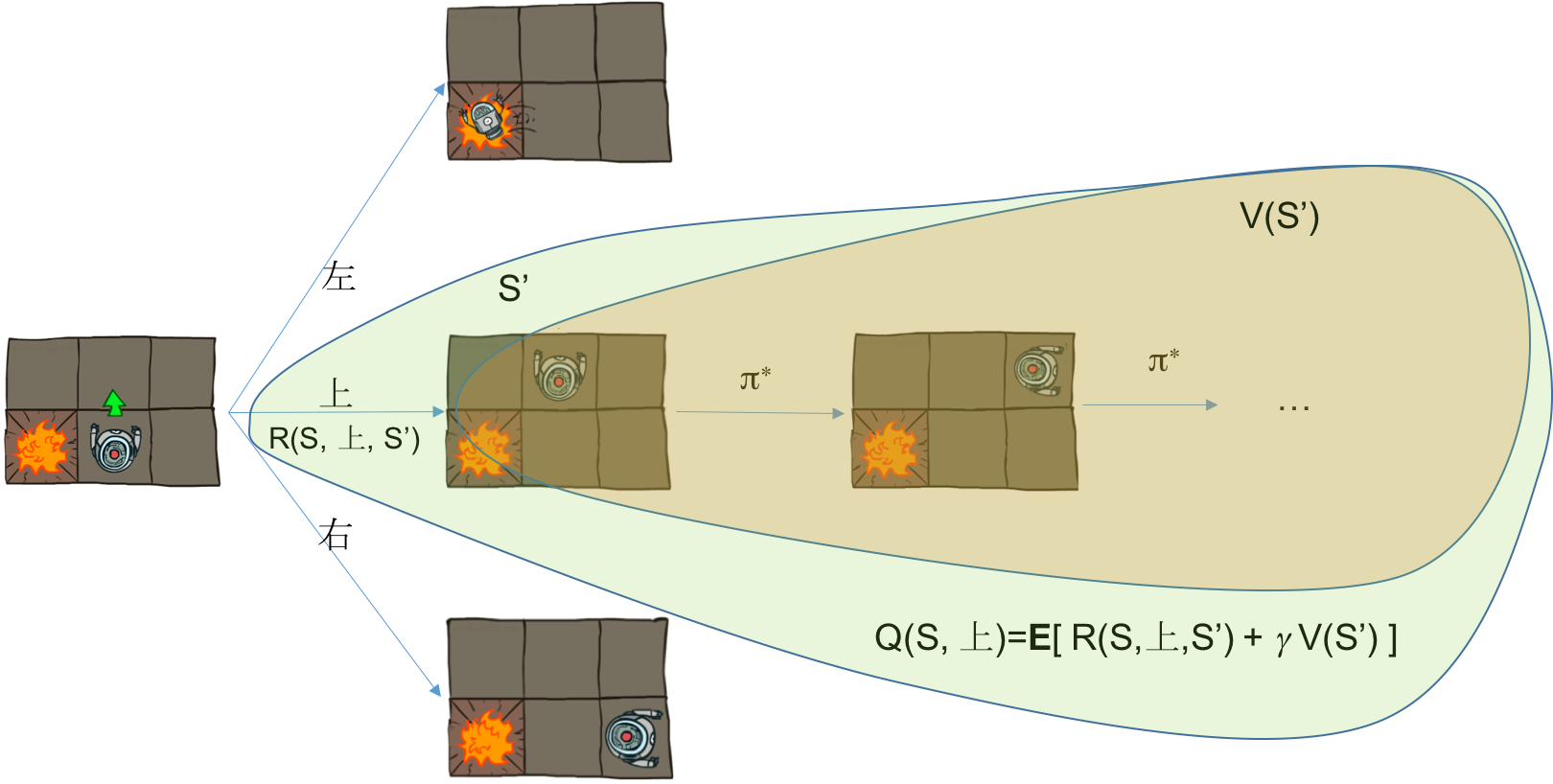$$Q(S, A) = \sum_{s'} P(s'| S, A)[ R(S, A, s') + \gamma V(s') ]$$

$$V(S) = \max_a Q(S, a)$$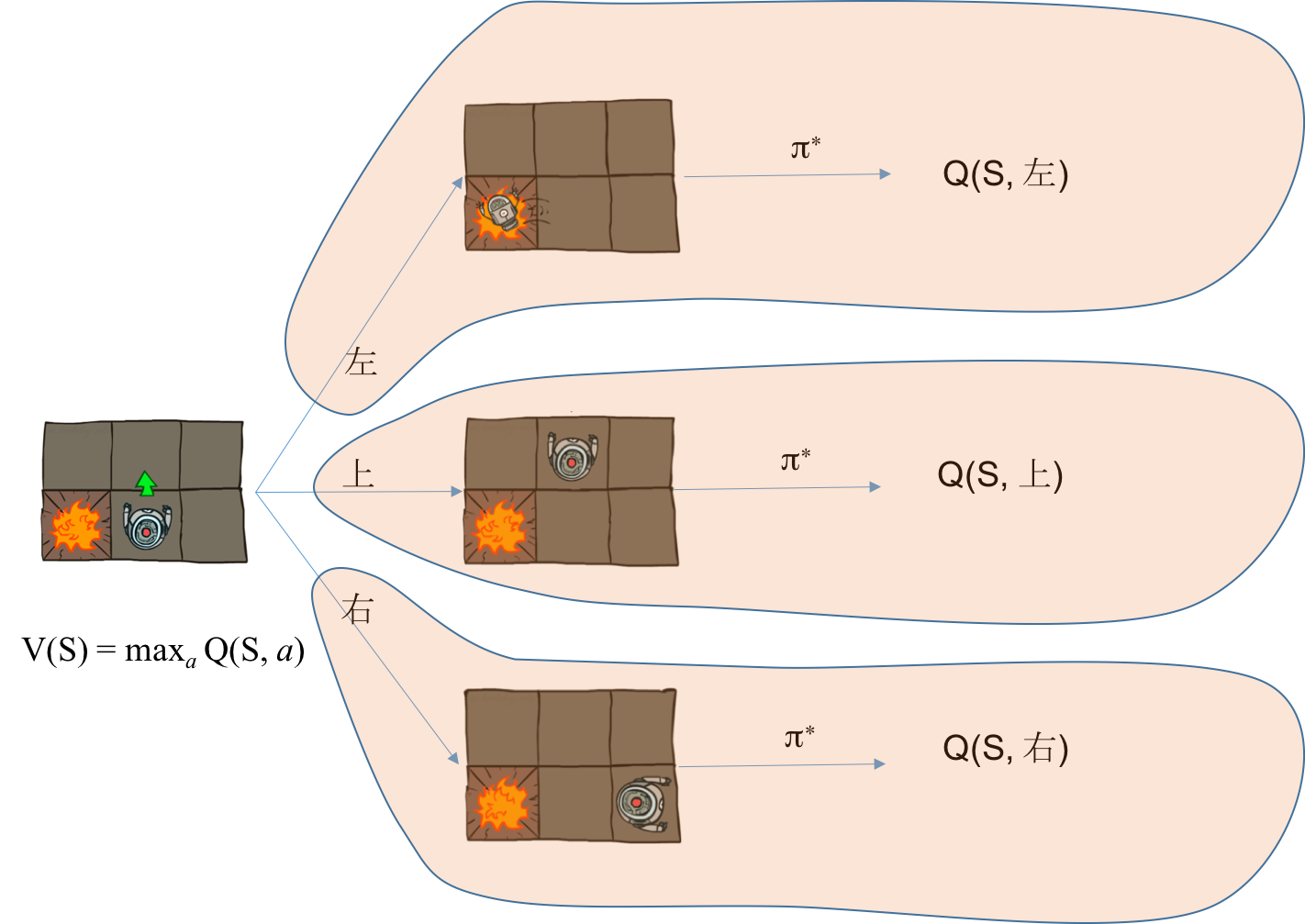$$Q^{\pi}(S, A) = E \left[ \sum_{t=1}^{\infty} \gamma^{t-1} R(S_t, A_t, S' _ t) | S_1=S, A_1=A, A_t \sim \pi \right]$$

### HJB方程

\begin{align} V(s) &= \max_a Q(s, a) \\ &= \max_a \sum_{s'} P(s'| s, a)[ R(s, a, s') + \gamma V(s') ] \end{align}

#### 确定性环境的HJB方程

$$V(s) = \max_a R(s, a, s') + \gamma V(s')$$

s'是在状态s下采用动作a后转移到的状态，在确定性迷宫问题中，考虑s=14的例子，HJB方程就是说(记住 V(15)=0)

$$V(14) = \max \{\gamma V(13), \gamma V(10), 1 \}$$

## 动态规划

### 值迭代

$$f(V) = \max_a \sum_{s'} P(s'| s, a)[ R(s, a, s') + \gamma V(s') ]$$

V是一个向量，包含多个元素，这个函数是一个多变量非线性函数。函数f的不动点是指满足方程$f(x) = x$的解。根据压缩映像原理，如果函数f是压缩映象，那么对任何初始值$V_0$，可以不断地应用函数f迭代下去$V_{k+1} = f(V_{k})$，$V_k$必收敛于函数f的不动点！而f的不动点就是满足HJB方程的状态值函数，所以有值迭代算法

1. 初始化$V_0(i)=0, i=1,2,...$
2. 利用HJB方程迭代 $V_{k+1}(s) = \max_a R(s, a, s') + \gamma V_{k}(s')$
3. 重复第2步直到收敛！

#### 压缩映像原理

$$d( f(x), f(y) ) \le \gamma d( x, y ), 0 < \gamma < 1$$

d是距离测度，也就是说在这个映射下，像的距离比原像的距离短，就像在压缩一样，所以叫做压缩映像(也说映射)。

$$|| x_{k} - x^ * || = || f(x_{k-1}) - f(x^ *) || \\ < \gamma || x_{k-1} - x^ * || < ... < \gamma^k ||x_0 - x^ * || \\ \rightarrow 0 (k \rightarrow \infty)$$

### 策略迭代

$$V^{\pi}(s) = \sum_{s'} P(s'| s, a)[ R(s, a, s') + \gamma V^{\pi}(s') ]$$

$$\pi'(s) = \arg\max_a Q^{\pi}(s, a)$$

1. 初始化一个策略$\pi$
2. 通过解线性方程计算策略$\pi$的值函数V和Q
3. 从Q函数中得到新的策略，更新到$\pi$中
4. 重复 2-3 直到策略收敛！

### 环境未知的问题

$$P(S'|S, A) = \frac{ N(S, A, S') }{\sum_{s'} N(S, A, s')} \\ R(S, A, S') = r$$

## 强化学习的应用

### 有注意力的图像识别系统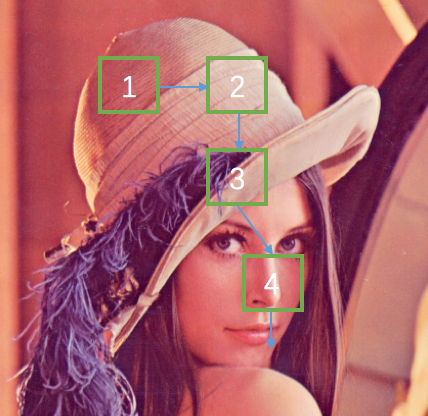## 思考与实践

1. 考虑如下图所示的MDP问题，S是初始状态，G是终止状态，对于非终止状态，每个状态可以采取两个动作：左或者右，每一步的回报都是-1。其中中间有一个状态，采取的动作和实际运动方向是相反的，也就是动作是向左，而实际运动是向右，其他两个状态正常。一个随机策略$\pi$定义如下：每一次都随机地以概率p选择动作“右”，以概率(1-p)选择动作“左”。试推导$V^{\pi}(S)$的最大值和此时的概率p的值。参考答案：p=0.59, V=-11.6。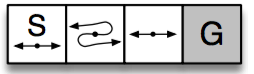1. 编程实现值迭代和策略迭代算法

0 1 2 ... 9
←·→ ←·→ ←·→ ←·→ 终点
import numpy as np

nS = 10
nA = 2  #不要改这个参数
Done = nS - 1
p0 = 0.1
p1 = 0.1
P = np.zeros((nS, nA, nS)) # 转移概率
R = np.zeros((nS, nA, nS)) - 1.0 # 回报都是-1
gamma = 1

# 环境构建
for s in range(nS):
if s == Done: # 终止态转移概率都为0
continue
for a in range(nA):
inc = a * 2 - 1 # 步长
P[s, a, s] += p0 # 不动
P[s, a, max(0, s - inc)] += p1 # 反方向
P[s, a, max(0, s + inc)] += 1 - p0 - p1 # 正常运动

V = np.zeros(nS)
# 值迭代
for it in range(1000):

## END
pass

print 'iteral steps:', it
print V

# 策略迭代

pi = np.zeros(nS, dtype=int) #初始策略全部往左

for it in range(100):
V = np.zeros(nS)
# 策略评估，解线性方程